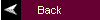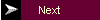MATLAB Tutorial5. Plotting

Commands covered:
plot
xlabel
ylabel
title
grid
axis
stem
subplot

The command most often used for plotting is plot, which creates linear plots of vectors and matrices; plot(t,y) plots the vector t on the x-axis versus vector y on the y-axis. There are options on the line type and the color of the plot which are obtained using plot(t,y,'option'). The linetype options are '-' solid line (default), '--' dashed line, '-.' dot dash line, ':' dotted line. The points in y can be left unconnected and delineated by a variety of symbols: + . * o x. The following colors are available options:

 r red b blue g green w white k black

For example, plot(t,y,'--') uses a dashed line, plot(t,y,'*') uses * at all the points defined in t and y without connecting the points, and plot(t,y,'g') uses a solid green line. The options can also be used together, for example, plot(t,y,'g:') plots a dotted green line.

To plot two or more graphs on the same set of axes, use the command plot(t1,y1,t2,y2), which plots y1 versus t1 and y2 versus t2.

To label your axes and give the plot a title, type

xlabel('time (sec)')
ylabel('step response')
title('My Plot')

grid

The problem that you will encounter most often when plotting functions is that MATLAB will scale the axes in a way that is different than you want them to appear. You can easily override the autoscaling of the axes by using the axis command after the plotting command:

axis([xmin xmax ymin ymax]);

where xmin, xmax, ymin, and ymax are numbers corresponding to the limits you desire for the axes. To return to the automatic scaling, simply type axis.

For discrete-time signals, use the command stem which plots each point with a small open circle and a straight line. To plot y[k] versus k, type stem(k,y). To obtain filled circles, as the plots appear in the textbook, use the command stem(k,y,'filled').

To plot more than one graph on the screen, use the command subplot(mnp) which partitions the screen into an mxn grid where p determines the position of the particular graph counting the upper left corner as p=1. For example,

subplot(211),semilogx(w,magdb);
subplot(212),semilogx(w,phase);

plots the bode plot with the log-magnitude plot on top and the phase plot below. Titles and labels can be inserted immediately after the appropriate semilogx command or plot command. To return to a full screen plot, type subplot(111).